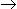# Number System (Test 4)

## Problem Solving And Reasoning : Mathematics Or Quantitative Aptitude

| Home | | Problem Solving And Reasoning | | Mathematics Or Quantitative Aptitude | | Number System |Number System
| Number System |
Q.1
The difference between a positive proper fraction and its reciprocal is 9/20. The fraction is:
A. 3/5
B. 3/10
C. 4/5
D. 4/3
Explaination / Solution:

 Let the required fraction be x. Then 1 - x = 9 x 201 - x2 = 9 x 2020 - 20x2 = 9x20x2 + 9x - 20 = 020x2 + 25x - 16x - 20 = 05x(4x + 5) - 4(4x + 5) = 0(4x + 5)(5x - 4) = 0

 x = 4 5

Workspace
Report
Q.2
On dividing a number by 56, we get 29 as remainder. On dividing the same number by 8, what will be the remainder ?
A. 4
B. 5
C. 6
D. 7
Explaination / Solution:

Formula: (Divisor*Quotient) + Remainder = Dividend.

Soln:

(56*Q)+29 = D -------(1)

D%8 = R -------------(2)

From equation(2),

((56*Q)+29)%8 = R.

=> Assume Q = 1.

=> (56+29)%8 = R.

=> 85%8 = R

=> 5 = R.

Workspace
Report
Q.3
If n is a natural number, then (6n2 + 6n) is always divisible by:
A. 6 only
B. 6 and 12 both
C. 12 only
D. by 18 only
Explaination / Solution:

(6n2 + 6n) = 6n(n + 1), which is always divisible by 6 and 12 both, since n(n + 1) is always even.

Workspace
Report
Q.4
107 x 107 + 93 x 93 = ?
A. 19578
B. 19418
C. 20098
D. 21908
E. None of these
Explaination / Solution:

 107 x 107 + 93 x 93 = (107)2 + (93)2 = (100 + 7)2 + (100 - 7)2 = 2 x [(100)2 + 72]       [Ref: (a + b)2 + (a - b)2 = 2(a2+ b2)] = 20098

Workspace
Report
Q.5
What will be remainder when (6767 + 67) is divided by 68 ?
A. 1
B. 63
C. 66
D. 67
Explaination / Solution:

(xn + 1) will be divisible by (x + 1) only when n is odd.(6767 + 1) will be divisible by (67 + 1)(6767 + 1) + 66, when divided by 68 will give 66 as remainder.

Workspace
Report
Q.6
On dividing a number by 5, we get 3 as remainder. What will the remainder when the square of the this number is divided by 5 ?
A. 0
B. 1
C. 2
D. 4
Explaination / Solution:

Let the number be x and on dividing x by 5, we get k as quotient and 3 as remainder.x = 5k + 3x2 = (5k + 3)2

= (25k2 + 30k + 9)

= 5(5k2 + 6k + 1) + 4On dividing x2 by 5, we get 4 as remainder.

Workspace
Report
Q.7
How many 3-digit numbers are completely divisible 6 ?
A. 149
B. 150
C. 151
D. 166
Explaination / Solution:

3-digit number divisible by 6 are: 102, 108, 114,... , 996

This is an A.P. in which a = 102, d = 6 and l = 996

Let the number of terms be n. Then tn = 996.a + (n - 1)d = 996102 + (n - 1) x 6 = 9966 x (n - 1) = 894(n - 1) = 149n = 150Number of terms = 150.

Workspace
Report
Q.8
How many natural numbers are there between 23 and 100 which are exactly divisible by 6 ?
A. 8
B. 11
C. 12
D. 13
E. None of these
Explaination / Solution:

Required numbers are 24, 30, 36, 42, ..., 96

This is an A.P. in which a = 24, d = 6 and l = 96

Let the number of terms in it be n.

Then tn = 96a + (n - 1)d = 9624 + (n - 1) x 6 = 96(n - 1) x 6 = 72(n - 1) = 12n = 13

Required number of numbers = 13.

Workspace
Report
Q.9
How many of the following numbers are divisible by 3 but not by 9 ? 2133, 2343, 3474, 4131, 5286, 5340, 6336, 7347, 8115, 9276
A. 5
B. 6
C. 7
D. None of these
Explaination / Solution:

Marking (/) those which are are divisible by 3 by not by 9 and the others by (X), by taking the sum of digits, we get:s

21339 (X)

234312 (/)

347418 (X)

41319 (X)

528621 (/)

534012 (/)

633618 (X)

734721 (/)

811515 (/)

927624 (/)

Required number of numbers = 6.

Workspace
Report
Q.10
 (963 + 476)2 + (963 - 476)2 = ? (963 x 963 + 476 x 476)
A. 1449
B. 497
C. 2
D. 4
E. None of these